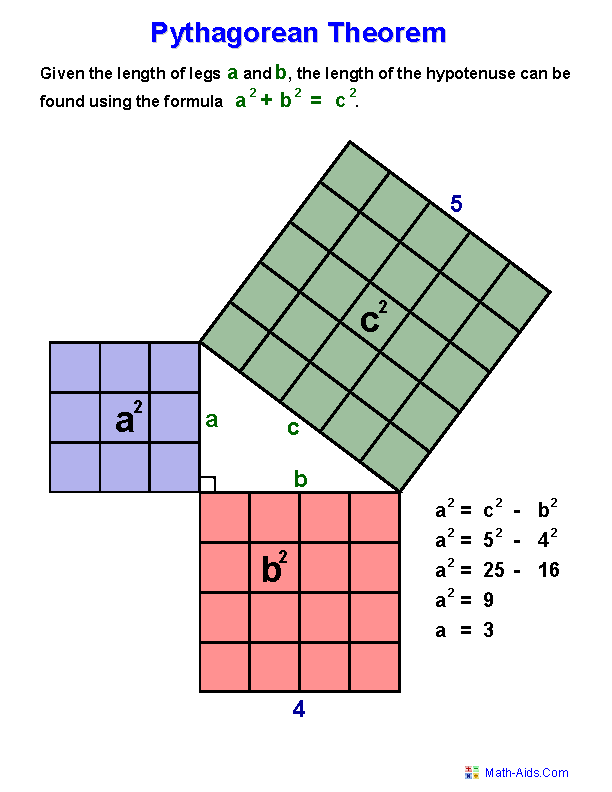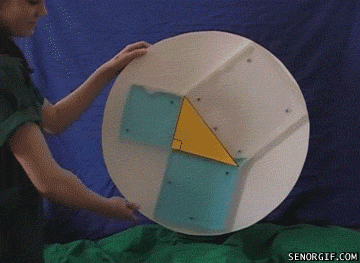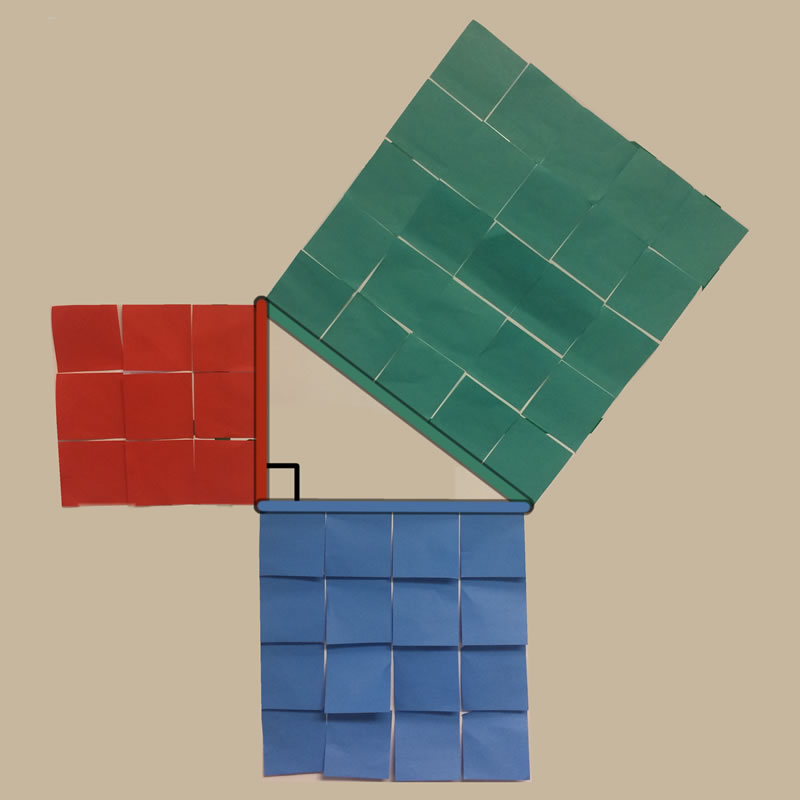# Geometry

Geometry

How to draw a regular hexagon (or decagon) using a ruler and a compassTry it out in your copybook or maybe start by drawing a perfect square using this fantastic geometry drawing website. Here’s another drawing program. You can install this app from the Google Chrome store.More Geometry-Pythagaros’ Theorem

In a right-angled triangle, the square on the hypotanuse (the long side) is equal in area to the sum of the squares on the other two sides (of the triangle).

## Pythagaros’ Theorem Exercises or Click hereFind N (use Pythagaros)Explanation without words. Use the slider.
Pythagaros with Post-It notes (animation-you will need 50)……..and with biscuits!!https://www.mathsisfun.com/pythagoras.html